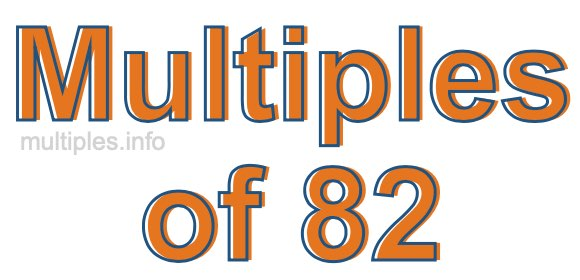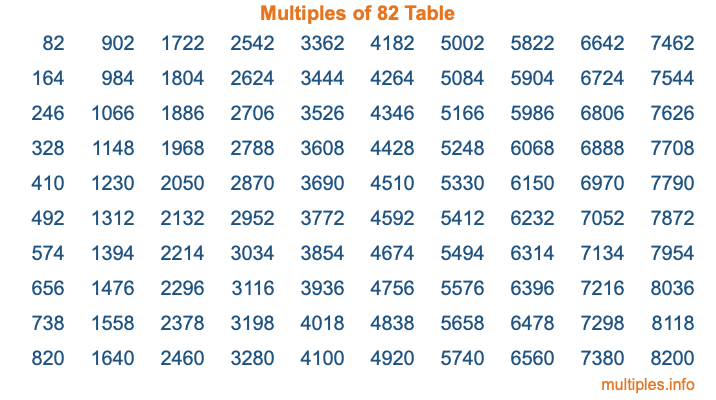Multiples of 82Welcome to the Multiples of 82 page. Here we will first teach you everything you will ever need to know about the multiples of 82, and then give you a study guide summary of everything we taught you to make sure you remember it all. Use this page to look up facts and learn information about the multiples of 82. This page will make you a multiples of eighty-two expert!

Definition of Multiples of 82
Multiples of 82 are all the numbers that when divided by 82 equal an integer. Each of the multiples of 82 are called a multiple. A multiple of 82 is created by multiplying 82 by an integer.

Therefore, to create a list of multiples of 82, you start with 1 multiplied by 82, then 2 multiplied by 82, then 3 multiplied by 82, and so on for as long as you want. Thus, the list of the first five multiples of 82 is 82, 164, 246, 328, and 410. To see a larger list of multiples of 82, see the printable image of Multiples of 82 further down on this page. We also have a category where you can choose any nth multiple of 82.

Multiples of 82 Checker
The Multiples of 82 Checker below checks to see if any number of your choice is a multiple of 82. In other words, it checks to see if there is any number (integer) that when multiplied by 82 will equal your number. To do that, we divide your number by 82. If the the quotient is an integer, then your number is a multiple of 82.

Is  a multiple of 82?

Least Common Multiple of 82 and ...
A Least Common Multiple (LCM) is the lowest multiple that two or more numbers have in common. This is also called the smallest common multiple or lowest common multiple and is useful to know when you are adding our subtracting fractions. Enter one or more numbers below (82 is already entered) to find the LCM.

Check out our LCM Calculator if you need more details about the Least Common Multiple or if you need the LCM for different numbers for adding and subtraction fractions.

nth Multiple of 82
As we stated above, 82 is the first multiple of 82, 164 is the second multiple of 82, 246 is the third multiple of 82, and so on. Enter a number below to find the nth multiple of 82.

th multiple of 82

Multiples of 82 vs Factors of 82
82 is a multiple of 82 and a factor of 82, but that is where the similarities end. All postive multiples of 82 are 82 or greater than 82. All positive factors of 82 are 82 or less than 82.

Below is the beginning list of multiples of 82 and the factors of 82 so you can compare:

Multiples of 82: 82, 164, 246, 328, 410, etc.

Factors of 82: 1, 2, 41, 82

As you can see, the multiples of 82 are all the numbers that you can divide by 82 to get a whole number. The factors of 82, on the other hand, are all the whole numbers that you can multiply by another whole number to get 82.

It's also interesting to note that if a number (x) is a factor of 82, then 82 will also be a multiple of that number (x).

Multiples of 82 vs Divisors of 82
The divisors of 82 are all the integers that 82 can be divided by evenly. Below is a list of the divisors of 82.

Divisors of 82: 1, 2, 41, 82

The interesting thing to note here is that if you take any multiple of 82 and divide it by a divisor of 82, you will see that the quotient is an integer.

Multiples of 82 Table
Below is an image of the first 100 multiples of 82 in a table. The table is in chronological order, column by column. The first column has the first ten multiples of 82, the second column has the next ten multiples of 82, and so on.The Multiples of 82 Table is also referred to as the 82 Times Table or Times Table of 82. You are welcome to print out our table for your studies.

Negative Multiples of 82
Although not often discussed or needed in math, it is worth mentioning that you can make a list of negative multiples of 82 by multiplying 82 by -1, then by -2, then by -3, and so on, to get the following list of negative multiples of 82:

-82, -164, -246, -328, -410, etc.

Multiples of 82 Summary
Below is a summary of important Multiples of 82 facts that we have discussed on this page. To retain the knowledge on this page, we recommend that you read through the summary and explain to yourself or a study partner why they hold true.

There are an infinite number of multiples of 82.

A multiple of 82 divided by 82 will equal a whole number.

82 divided by a factor of 82 equals a divisor of 82.

The nth multiple of 82 is n times 82.

The largest factor of 82 is equal to the first positive multiple of 82.

82 is a multiple of every factor of 82.

82 is a multiple of 82.

A multiple of 82 divided by a divisor of 82 equals an integer.

82 divided by a divisor of 82 equals a factor of 82.

Any integer times 82 will equal a multiple of 82.

Multiples of a Number
Here you can get the multiples of another number, all with the same attention to detail as we did for multiples of 82 on this page.

Multiples of
Multiples of 83
Did you find our page about multiples of eighty-two educational? Do you want more knowledge? Check out the multiples of the next number on our list!